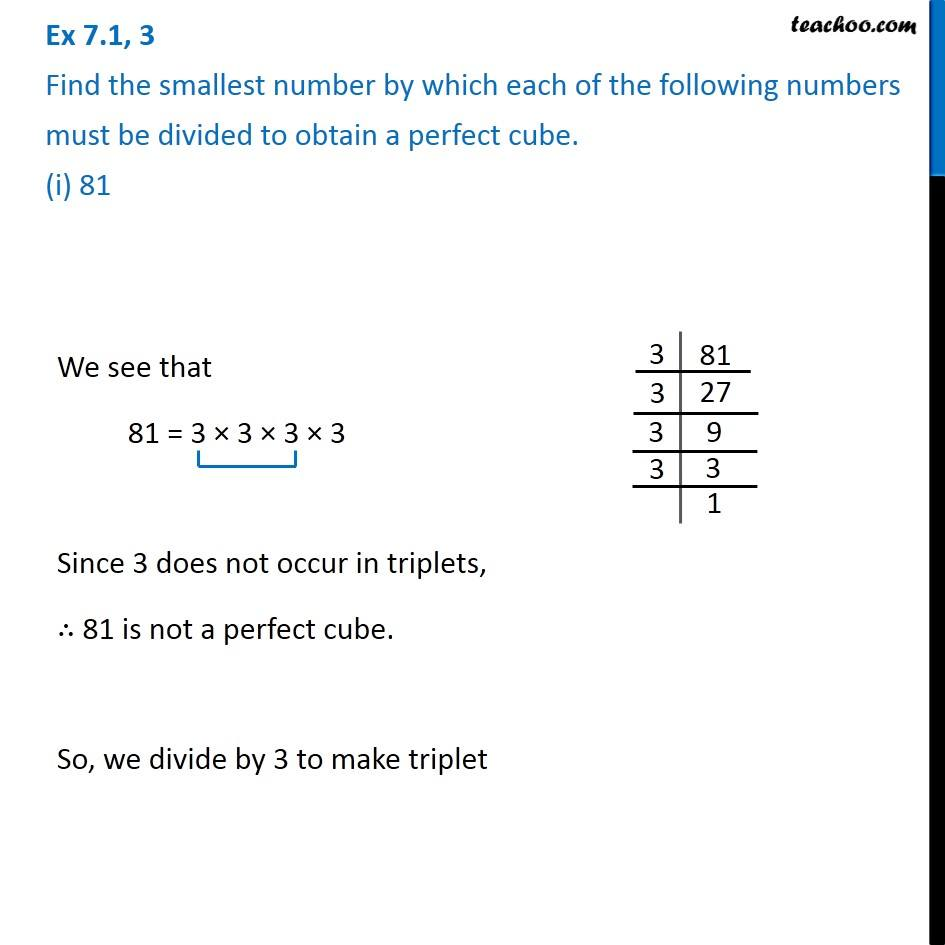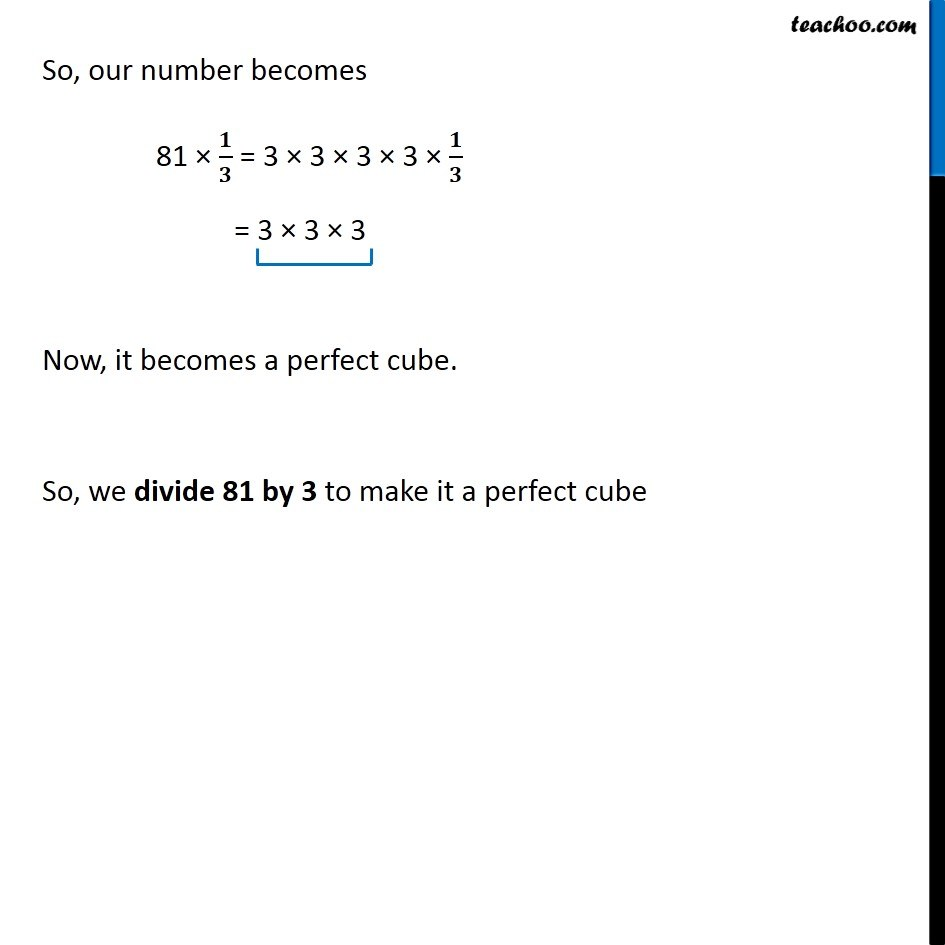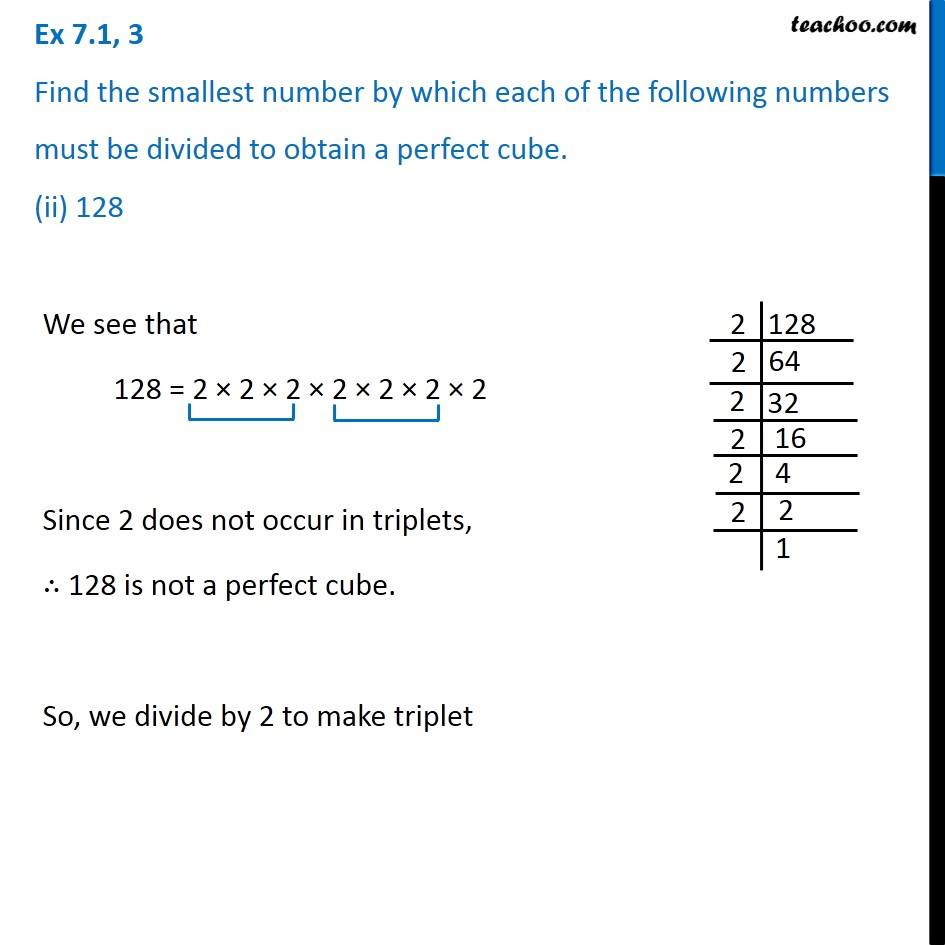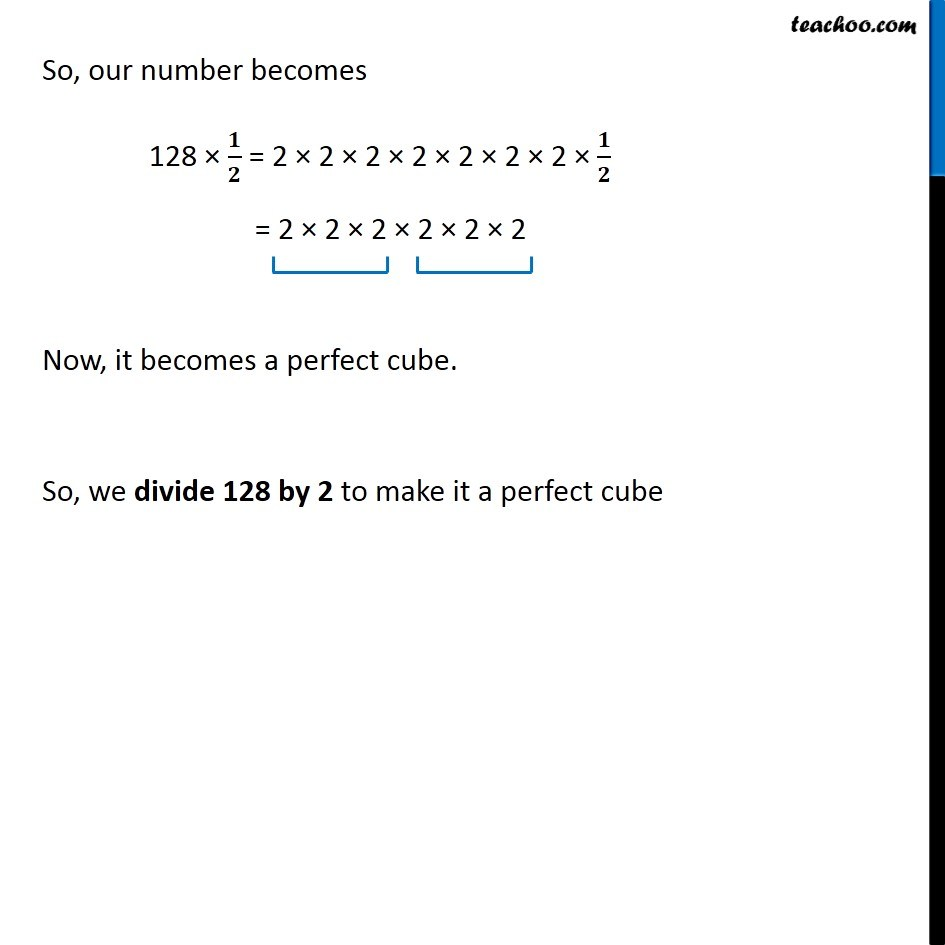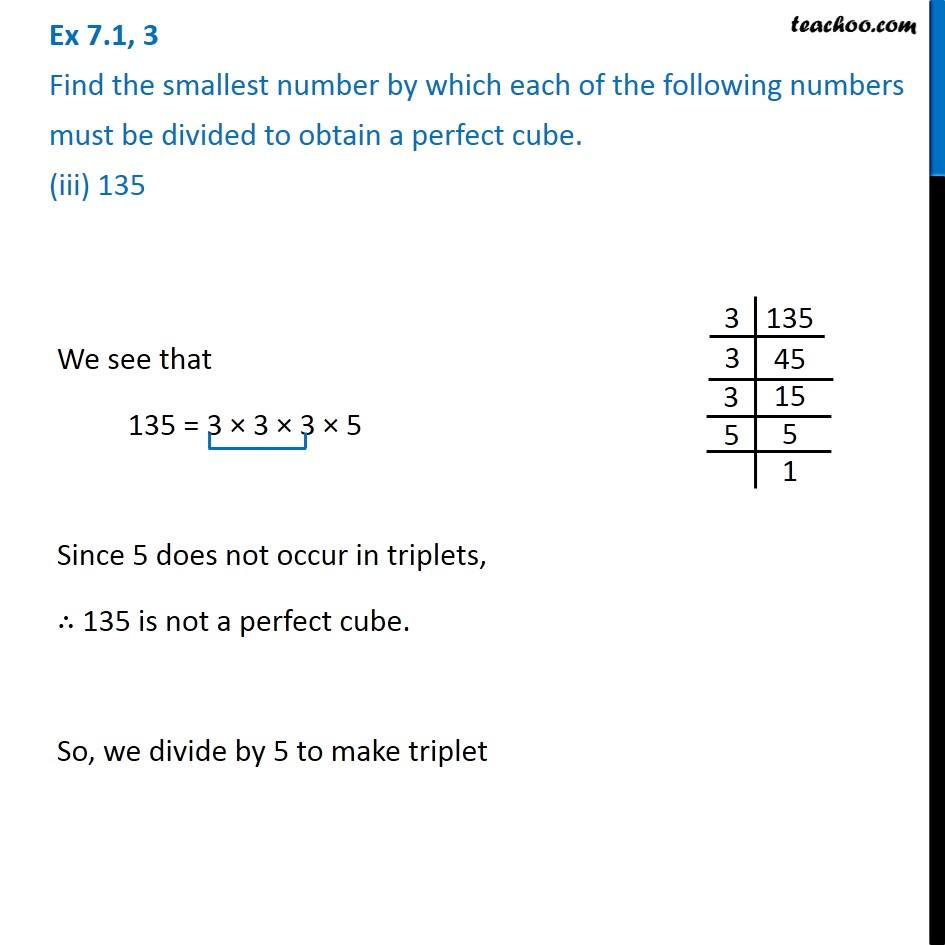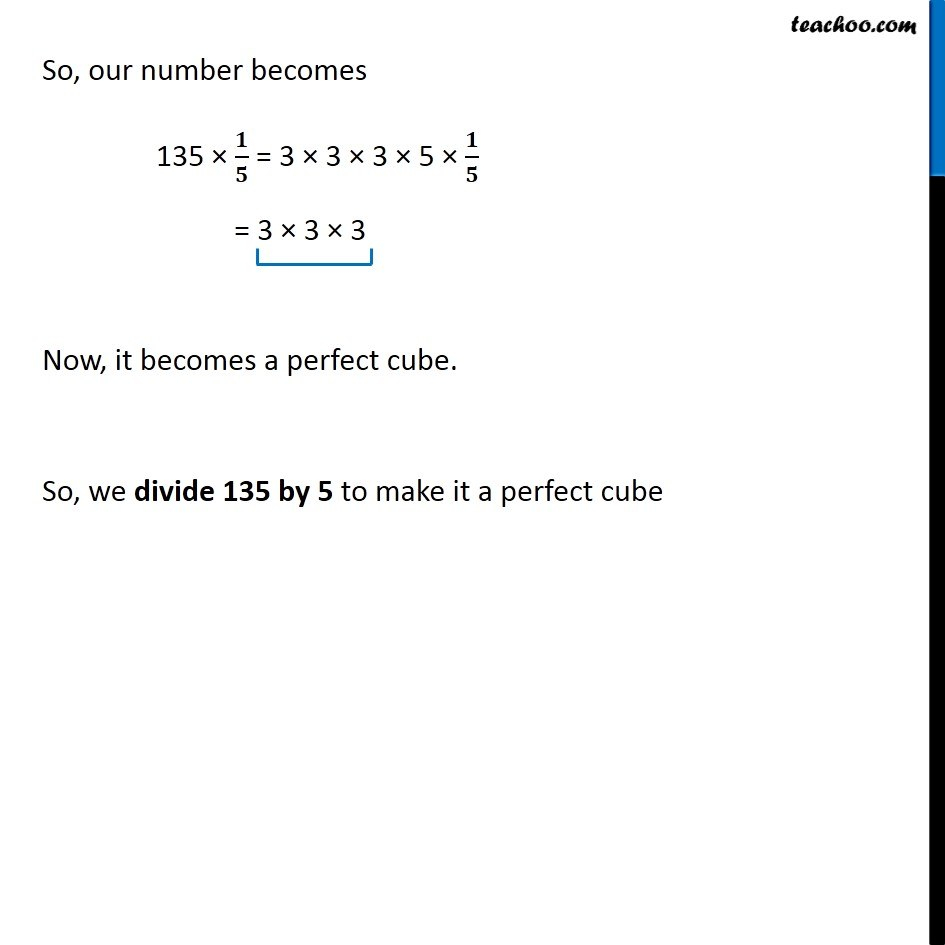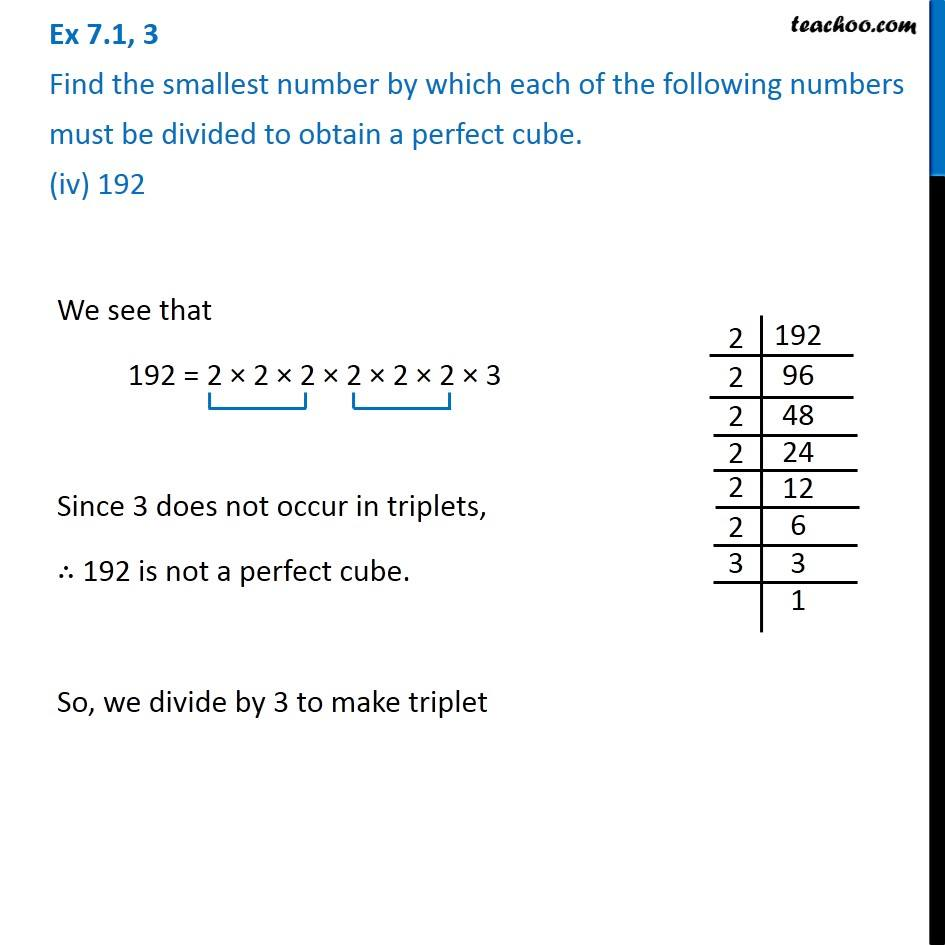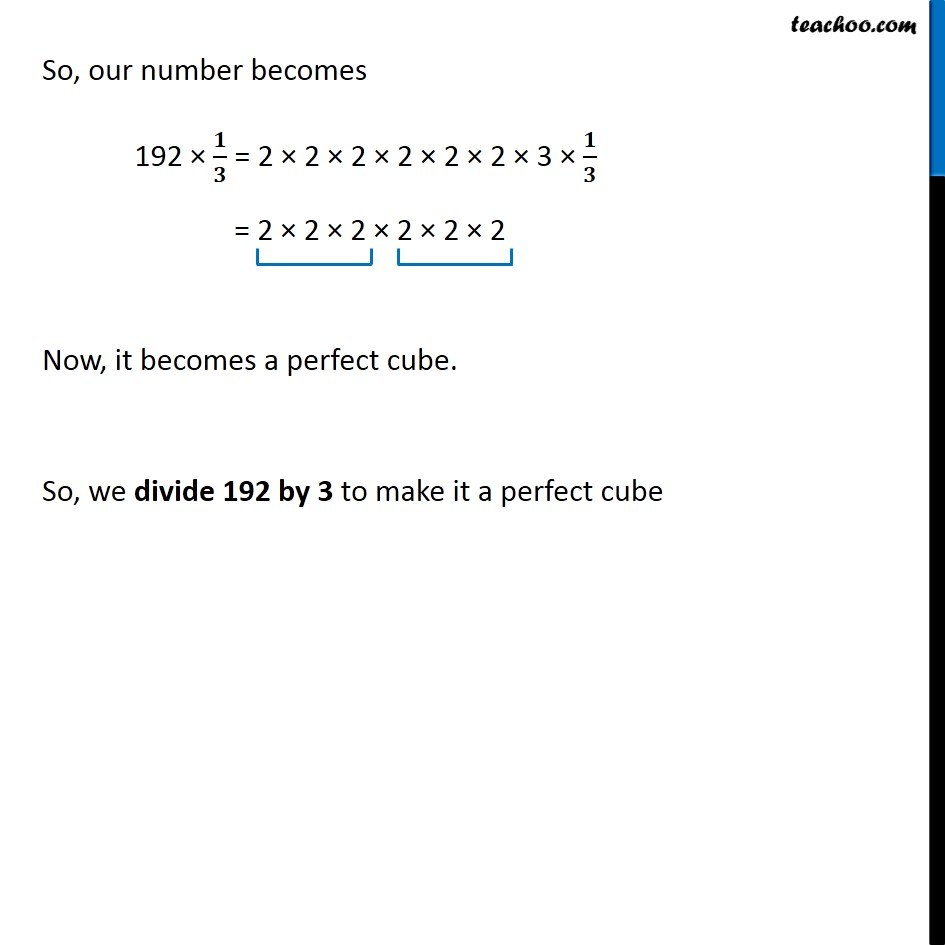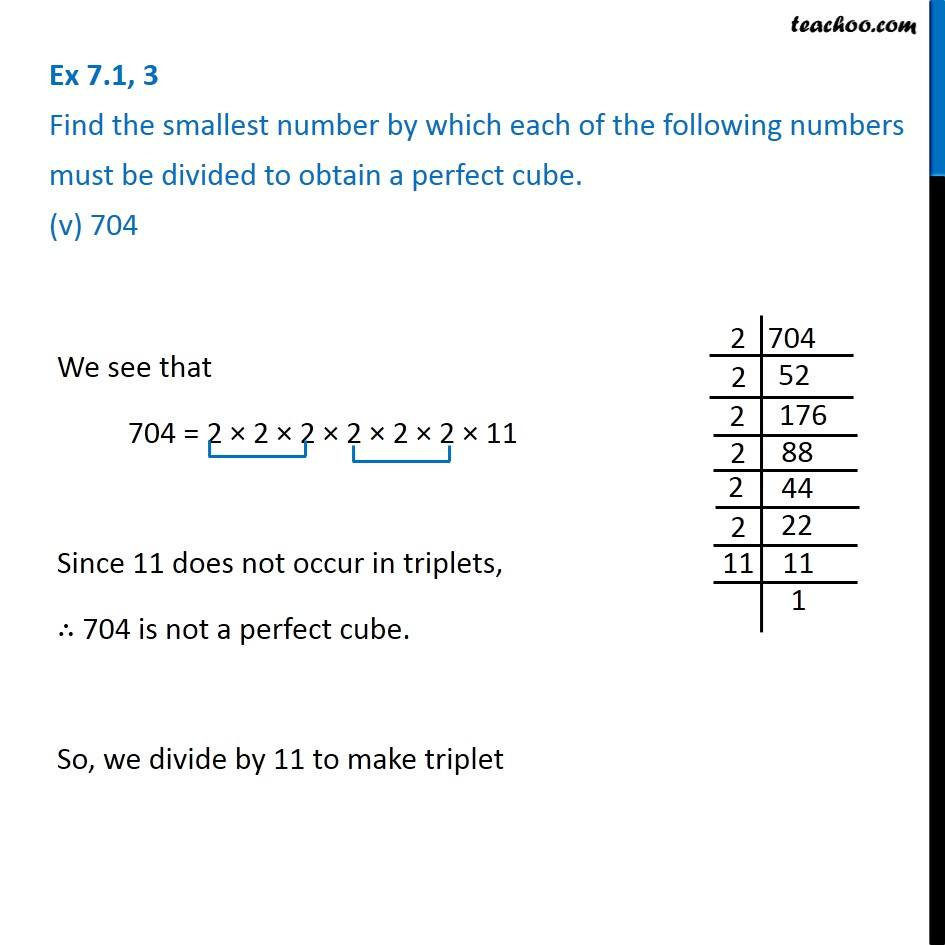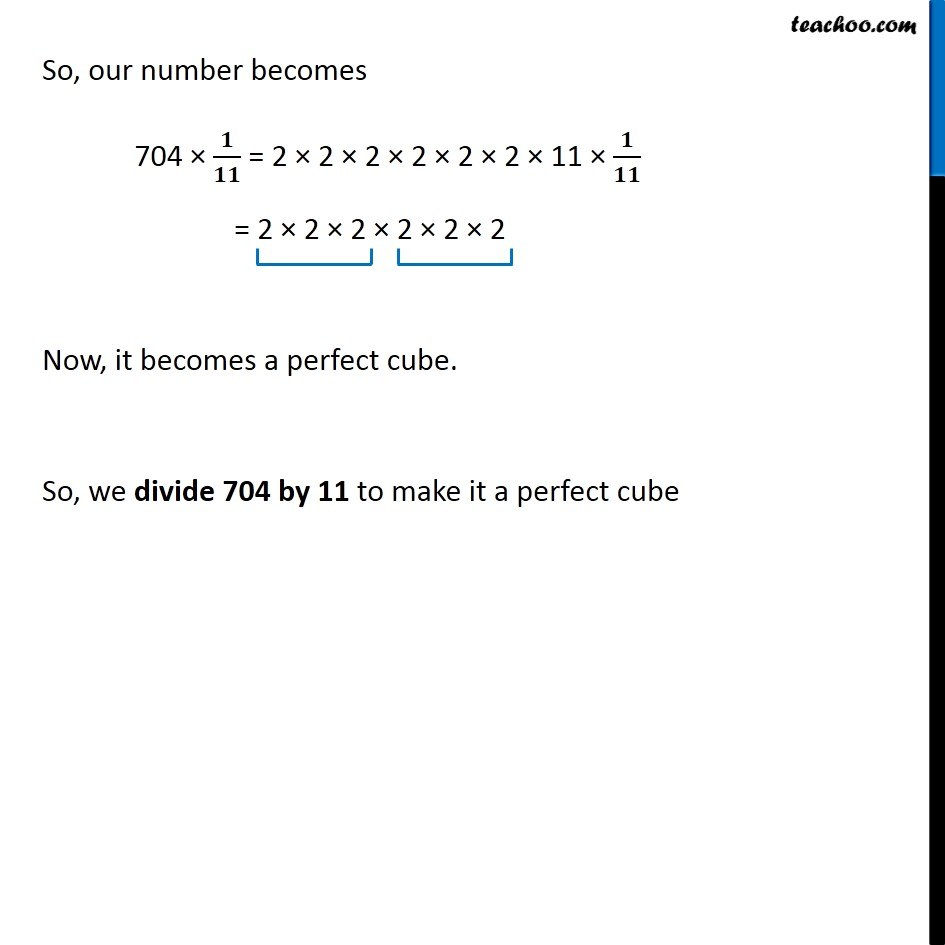Subscribe to our Youtube Channel - https://you.tube/teachoo

1. Chapter 7 Class 8 Cubes and Cube Roots
2. Serial order wise
3. Ex 7.1

Transcript

Ex 7.1, 3 Find the smallest number by which each of the following numbers must be divided to obtain a perfect cube. (i) 81 We see that 81 = 3 × 3 × 3 × 3 Since 3 does not occur in triplets, ∴ 81 is not a perfect cube. So, we divide by 3 to make triplet So, our number becomes 81 × 𝟏/𝟑 = 3 × 3 × 3 × 3 × 𝟏/𝟑 = 3 × 3 × 3 Now, it becomes a perfect cube. So, we divide 81 by 3 to make it a perfect cube Ex 7.1, 3 Find the smallest number by which each of the following numbers must be divided to obtain a perfect cube. (ii) 128We see that 128 = 2 × 2 × 2 × 2 × 2 × 2 × 2 Since 2 does not occur in triplets, ∴ 128 is not a perfect cube. So, we divide by 2 to make triplet So, our number becomes 128 × 𝟏/𝟐 = 2 × 2 × 2 × 2 × 2 × 2 × 2 × 𝟏/𝟐 = 2 × 2 × 2 × 2 × 2 × 2 Now, it becomes a perfect cube. So, we divide 128 by 2 to make it a perfect cube Ex 7.1, 3 Find the smallest number by which each of the following numbers must be divided to obtain a perfect cube. (iii) 135 We see that 135 = 3 × 3 × 3 × 5 Since 5 does not occur in triplets, ∴ 135 is not a perfect cube. So, we divide by 5 to make triplet So, our number becomes 135 × 𝟏/𝟓 = 3 × 3 × 3 × 5 × 𝟏/𝟓 = 3 × 3 × 3 Now, it becomes a perfect cube. So, we divide 135 by 5 to make it a perfect cube Ex 7.1, 3 Find the smallest number by which each of the following numbers must be divided to obtain a perfect cube. (iv) 192We see that 192 = 2 × 2 × 2 × 2 × 2 × 2 × 3 Since 3 does not occur in triplets, ∴ 192 is not a perfect cube. So, we divide by 3 to make triplet So, our number becomes 192 × 𝟏/𝟑 = 2 × 2 × 2 × 2 × 2 × 2 × 3 × 𝟏/𝟑 = 2 × 2 × 2 × 2 × 2 × 2 Now, it becomes a perfect cube. So, we divide 192 by 3 to make it a perfect cube Ex 7.1, 3 Find the smallest number by which each of the following numbers must be divided to obtain a perfect cube. (v) 704 We see that 704 = 2 × 2 × 2 × 2 × 2 × 2 × 11 Since 11 does not occur in triplets, ∴ 704 is not a perfect cube. So, we divide by 11 to make triplet So, our number becomes 704 × 𝟏/𝟏𝟏 = 2 × 2 × 2 × 2 × 2 × 2 × 11 × 𝟏/𝟏𝟏 = 2 × 2 × 2 × 2 × 2 × 2 Now, it becomes a perfect cube. So, we divide 704 by 11 to make it a perfect cube

Ex 7.1